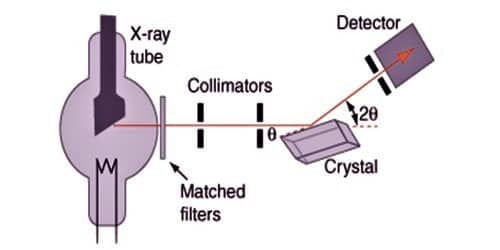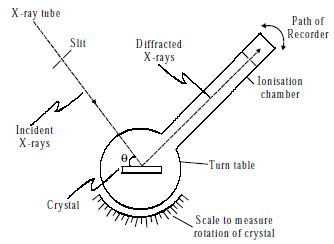Chemistry

# Explain Bragg’s Spectrometer MethodBragg’s spectrometer method is one of the important methods for studying crystals using X-rays. The apparatus consists of an X-ray tube from which a narrow beam of X-rays is allowed to fall on the crystal mounted on a rotating table. The rotating table is provided with scale and vernier, from which the angle of incidence, θ can be measured.Fig: X-ray spectrometer

An arm which is rotating about the same axis as the crystal table carries an ionization chamber. The rays reflected from the crystal enter into the ionization chamber and ionize the gas present inside. Due to the ionization, the current is produced which is measured by the electrometer. The current of ionization is a direct measure of the intensity of reflected beam from the crystal. For different angles of incidence, the corresponding ionization current is measured from the electrometer. These values are plotted in the form of the graph.

For sodium chloride, the maximum reflection or peaks for 100 plane occurred at θ = 5.9°, 11.85°, and 18.15°. The sines of these angles are 0.103, 0.205 and 0.312 which are in the ratio 1:2:3. These peaks represent the first, second and third order reflections. The ratio confirms the correctness of Bragg’s equation.

The first ionization spectrometer intended and constructed by William Henry Bragg in 1912-13, used to calculate variations in scattering angles of crystals in order to establish their structures.

Bragg’s equation is nλ = 2d sinθ

Where; n is the order of reflection

λ is the wavelength of X-rays

d is the interplanar distance in the crystal

θ is the angle of reflection.

The principle of Bragg’s law is applied in the structure of instruments such as Bragg spectrometer, which is often used to study the structure of crystals and molecules. Bragg’s Law can simply be derived by considering the conditions obligatory to make the phases of the beams coincide when the incident angle equals and reflecting angle.

To obtain almost monochromatic x-rays, an x-ray tube is used to create characteristic x-rays. The Braggs used crystals in the reflection geometry to evaluate the intensity and wavelengths of X-rays (spectra) generated by different materials. Their apparatus for characterizing X-ray spectra was the Bragg spectrometer.